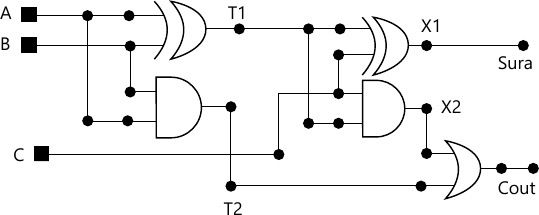MORE IN Digital Electronics & Logic Design
SPPU Information Technology (Semester 3)
Digital Electronics & Logic Design
December 2015
Total marks: --
Total time: --
INSTRUCTIONS
(1) Assume appropriate data and state your reasons
(2) Marks are given to the right of every question
(3) Draw neat diagrams wherever necessary

Solve any one question from Q1 and Q2
1 (a) Convert the following numbers, show all steps:
i) (2598.675)10 = ( )16
ii) (110101.101010)2 = ( )8
iii) (A72E)16 = ( )8.
6 M
1 (b) Draw and explain CMOS Non-inverting buffer.
6 M

2 (a) Design full Subtractor using Decoder IC 74138.
6 M
2 (b) Explain the following TTL characteristics.
i) Noise Immunity
ii) High level input voltage (VIH)
iii) Figure of Merit
6 M

Solve any one question from Q3 and Q4
3 (a) What is race around condition? Explain with the help of timing diagram. How is it removed using Flip-Flop.
7 M
3 (b) Draw and explain 3-bit ring counter.
6 M

4 (a) Design a sequence generator to generate the sequence .....10110..... using IC 74194.
7 M
4 (b) Design MOD-11 UP counter using IC 74191.
6 M

Solve any one question from Q5 and Q6
5 (a) Design the following functions using PLA.
F1=∑m (1, 3, 5)
F2=∑m (5, 6, 7).
6 M
5 (b) Draw and explain block diagram of CPLD.
6 M

6 (a) Explain the difference between CPLD and FPGA.
6 M
6 (b) Design 4:1 Multiplexer using PAL.
6 M

Solve any one question from Q7 and Q8
7 (a) Explain the difference between data flow and behavioural modelling.
7 M
7 (b) Write entity and architecture of D Flip-flop with clear input using behavioural modelling.
6 M

8 (a) Write entity and architecture of following circuit using structural modelling.7 M
8 (b) What is VHDL? Draw the structure of VHDL module and explain various components of it.
6 M

More question papers from Digital Electronics & Logic Design